Finding General Solutions

Chapter 3 Class 11 Trigonometric Functions
Concept wise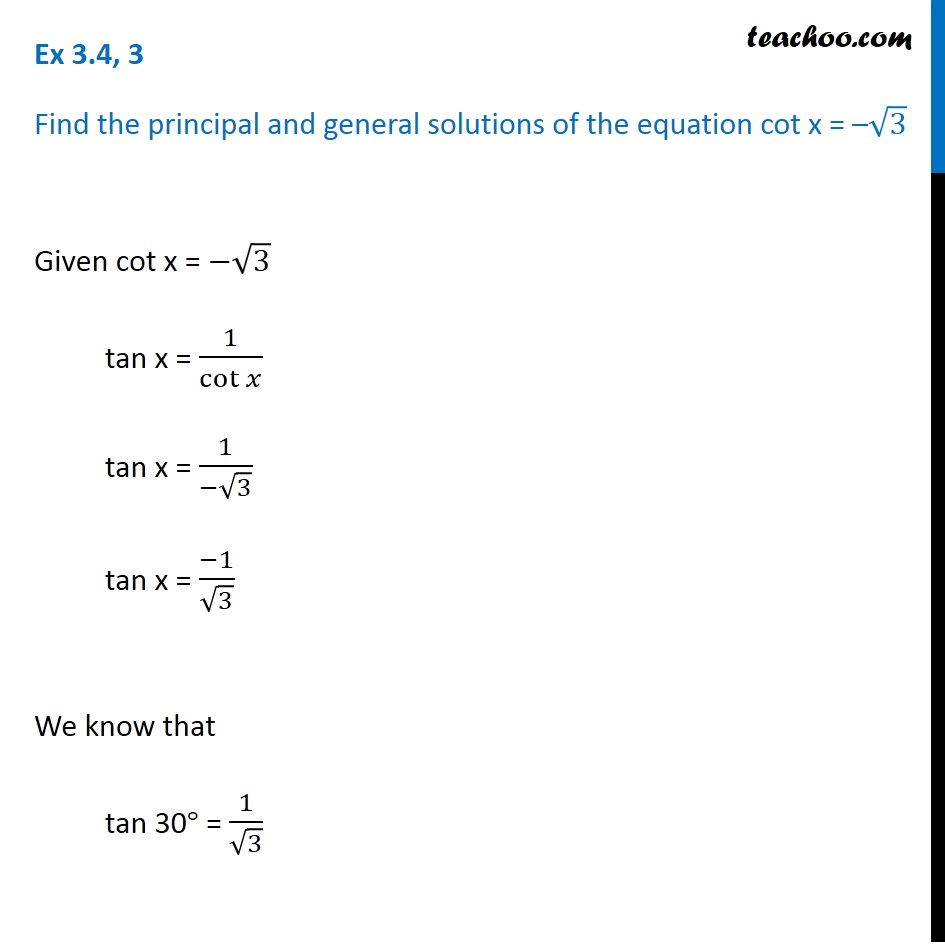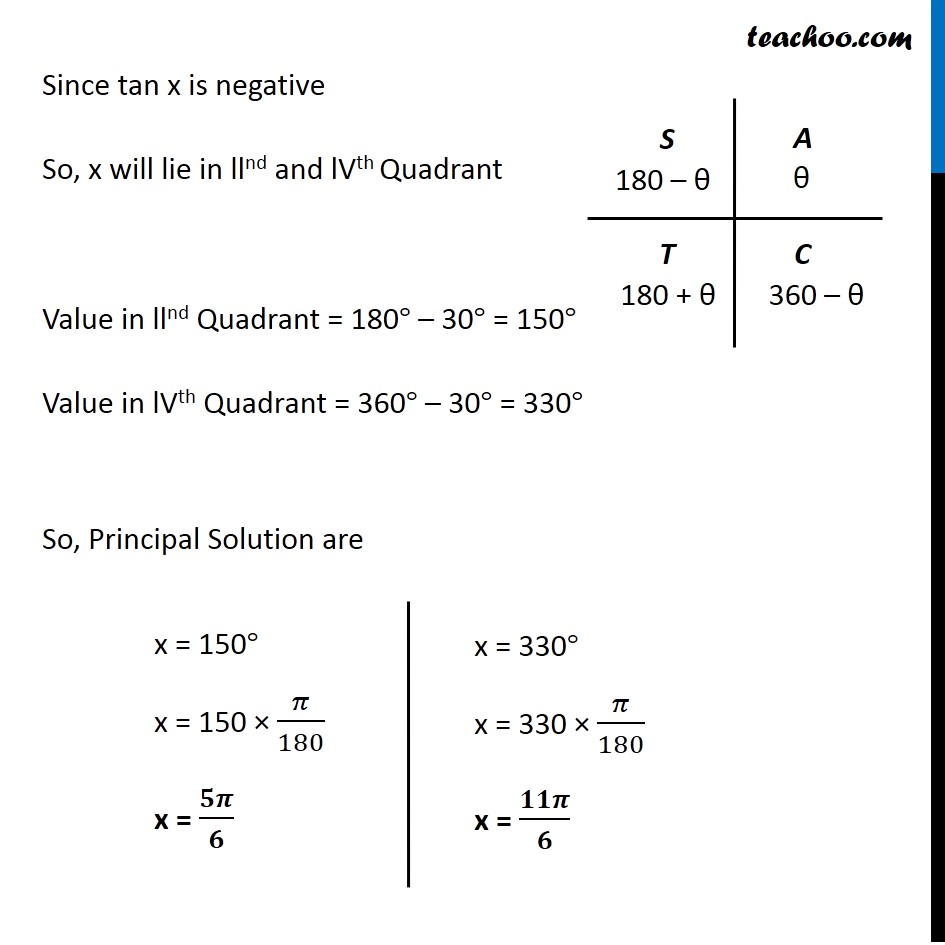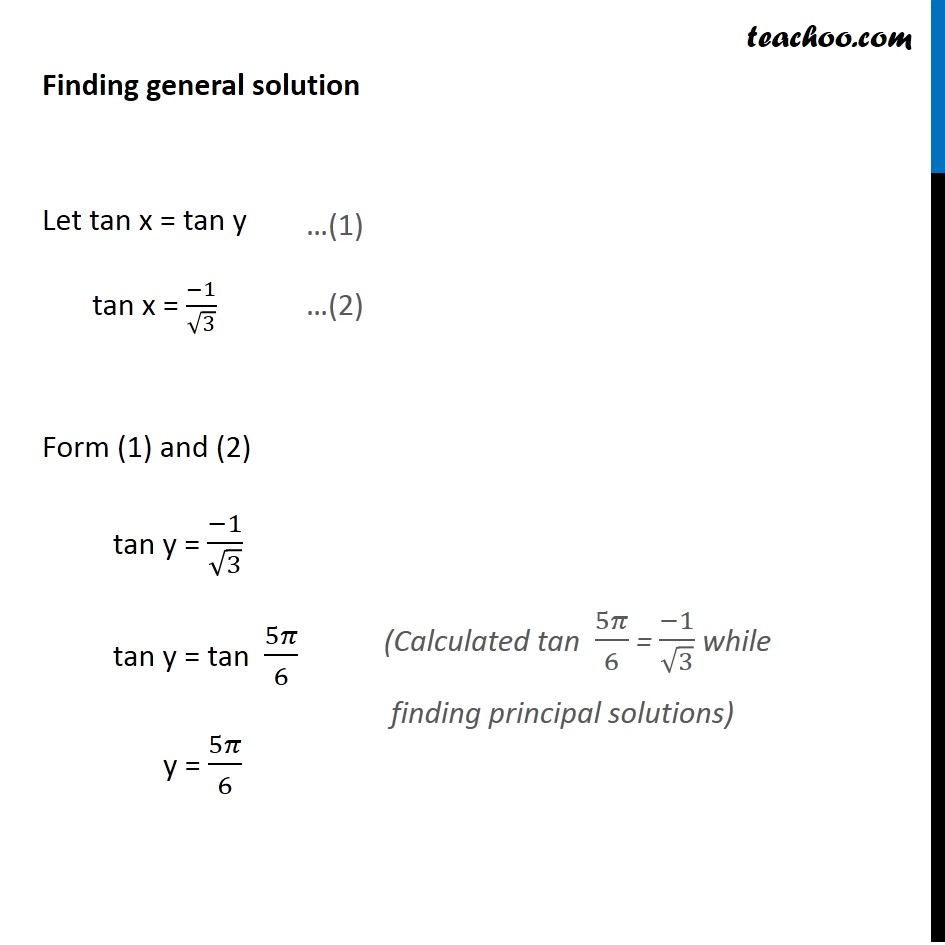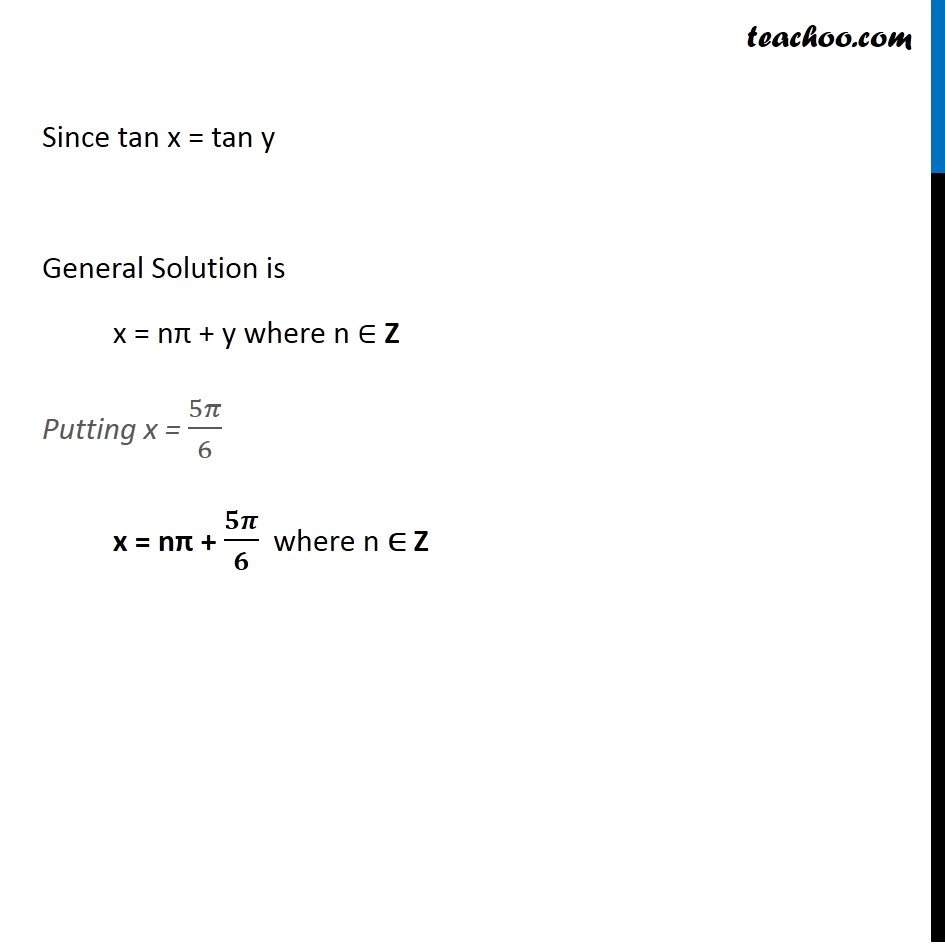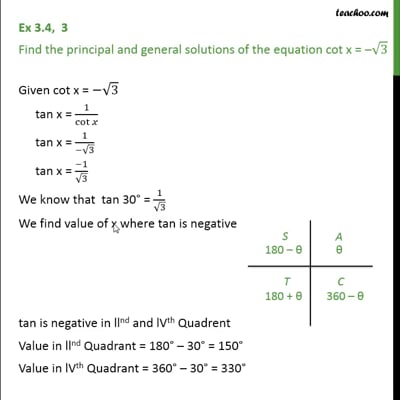This video is only available for Teachoo black users

Introducing your new favourite teacher - Teachoo Black, at only ₹83 per month

### Transcript

Ex 3.4, 3 Find the principal and general solutions of the equation cot x = –√3 Given cot x = −√3 tan x = 1/cot⁡𝑥 tan x = 1/(−√3) tan x = (−1)/√3 We know that tan 30° = 1/√3 Since tan x is negative So, x will lie in llnd and lVth Quadrant Value in llnd Quadrant = 180° – 30° = 150° Value in lVth Quadrant = 360° – 30° = 330° So, Principal Solution are x = 150° x = 150 × 𝜋/180 x = 𝟓𝝅/𝟔 x = 330° x = 330 × 𝜋/180 x = 𝟏𝟏𝝅/𝟔 Finding general solution Let tan x = tan y tan x = (−1)/√3 Form (1) and (2) tan y = (−1)/√3 tan y = tan 5𝜋/6 y = 5𝜋/6 (Calculated tan 5𝜋/6 = (−1)/√3 while finding principal solutions) Since tan x = tan y General Solution is x = nπ + y where n ∈ Z Putting x = 5𝜋/6 x = nπ + 𝟓𝝅/𝟔 where n ∈ Z